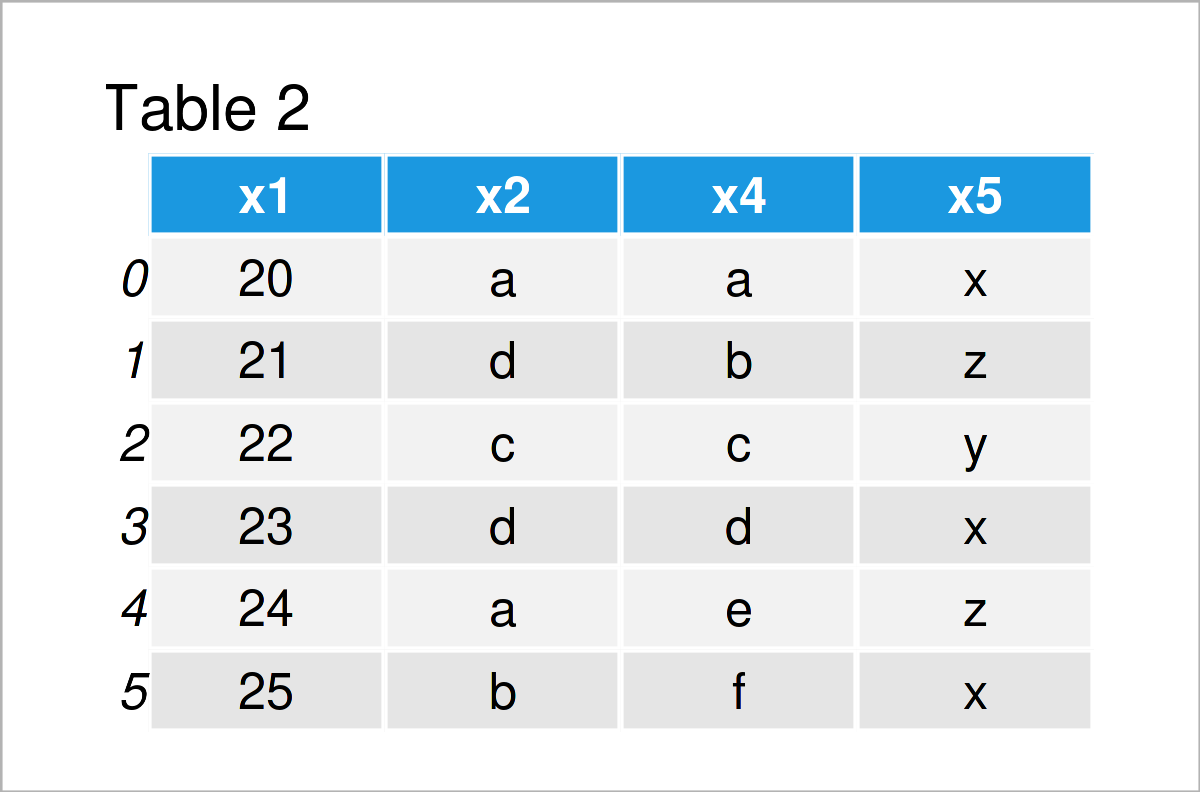# Drop pandas DataFrame Column by Index in Python (2 Examples)

In this Python article you’ll learn how to delete a pandas DataFrame column by index.

The article will consist of two examples for the removal of a pandas DataFrame variable by index. To be more specific, the tutorial contains the following content blocks:

Let’s dive into it…

## Example Data & Add-On Libraries

In order to use the functions of the pandas library, we first need to import pandas:

`import pandas as pd                                       # Load pandas library`

Next, we’ll also have to create some exemplifying data:

```data = pd.DataFrame({'x1':range(20, 26),                  # Create pandas DataFrame
'x2':['a', 'd', 'c', 'd', 'a', 'b'],
'x3':range(27, 21, - 1),
'x4':['a', 'b', 'c', 'd', 'e', 'f'],
'x5':['x', 'z', 'y', 'x', 'z', 'x']})
print(data)                                               # Print pandas DataFrame```As you can see based on Table 1, our example data is a pandas DataFrame containing six rows and five columns.

## Example 1: Drop One pandas DataFrame Column by Index

Example 1 demonstrates how to remove a single variable by its index position from a pandas DataFrame in Python.

For this, we can use the drop function as shown in the following Python code:

```data_new1 = data.drop(data.columns, axis = 1)          # Drop single variable
print(data_new1)                                          # Print updated DataFrame```Table 2 shows the output of the previously shown code – A pandas DataFrame subset where the column x3 has been deleted.

## Example 2: Drop Multiple pandas DataFrame Columns by Index

Example 2 illustrates how to drop multiple columns by index from a pandas DataFrame.

To achieve this, we first have to specify all index locations that we want to exclude in a list object:

```drop_list = [1, 2, 4]                                     # Create list of indices
print(drop_list)                                          # Print list of indices
# [1, 2, 4]```

Now, we can use this list within the drop function to remove all columns at these index locations:

```data_new2 = data.drop(data.columns[drop_list], axis = 1)  # Drop multiple variables
print(data_new2)                                          # Print updated DataFrame```As shown in Table 3, we have created another subset of our input data set where the columns x2, x3, and x5 have been dropped.

## Video & Further Resources

I have recently published a video on my YouTube channel, which illustrates the Python programming code of this article. You can find the video below:

Furthermore, you may read the other tutorials on my website. You can find some tutorials below.

This post has explained how to remove a pandas DataFrame variable by index in the Python programming language. In case you have further questions, don’t hesitate to let me know in the comments section. Furthermore, please subscribe to my email newsletter to get updates on the newest tutorials.

Subscribe to the Statistics Globe Newsletter